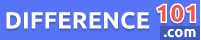Average

The average is the arithmetic mean of a set of numbers, the most common form of which is the arithmetic mean of a set of observations. It is defined as the sum of the numbers in the set divided by the number of items in the set. The arithmetic mean of a set of numbers x1, x2, …, xn is denoted by x̄ and is given by x̄ = (x1 + x2 + … + xn)/n.